# 强化学习101: Q-Learning - 从人工智障到人工智能## 1 强化学习初见

### 1.1 引入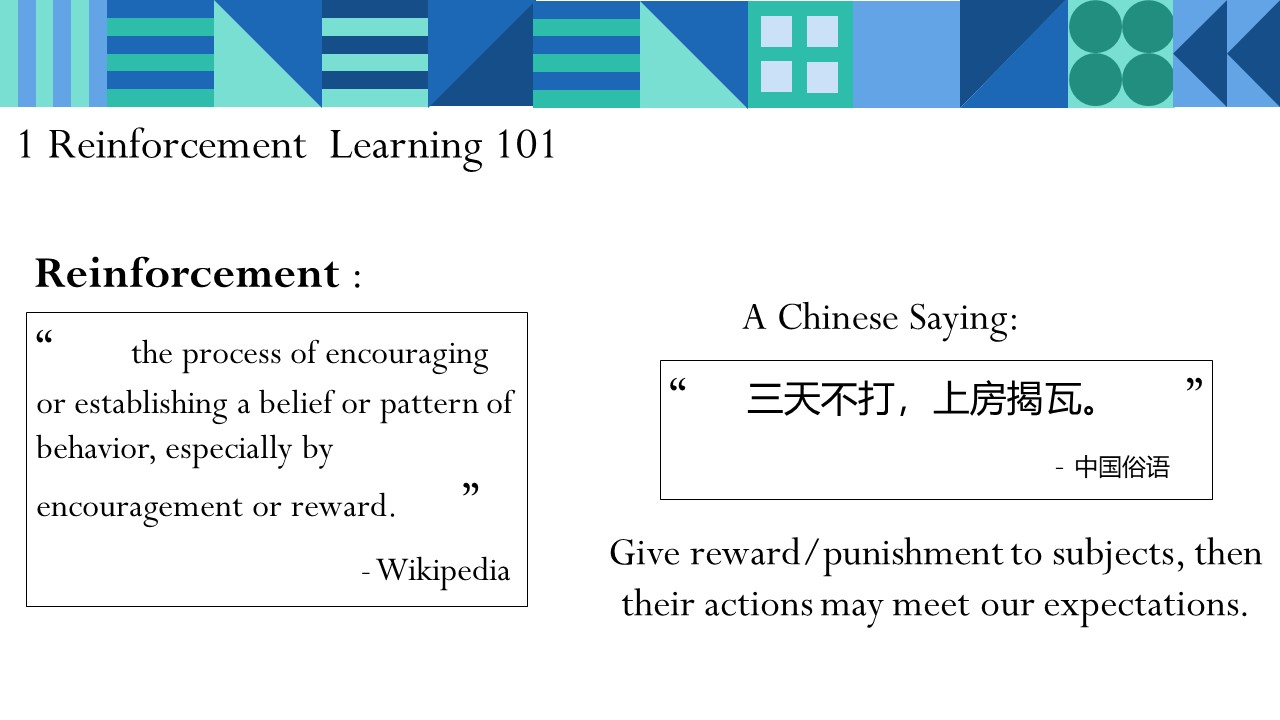“强化学习”一词的英文表述为Reinforcement Learning。也有人把它表述成为“增强学习”。既然这个词是舶来的，让我们先看看Reinforcement的英文表述：

the process of encouraging or establishing a belief or pattern of behavior, especially by encouragement or reward.

### 1.2 俄罗斯方块与玩家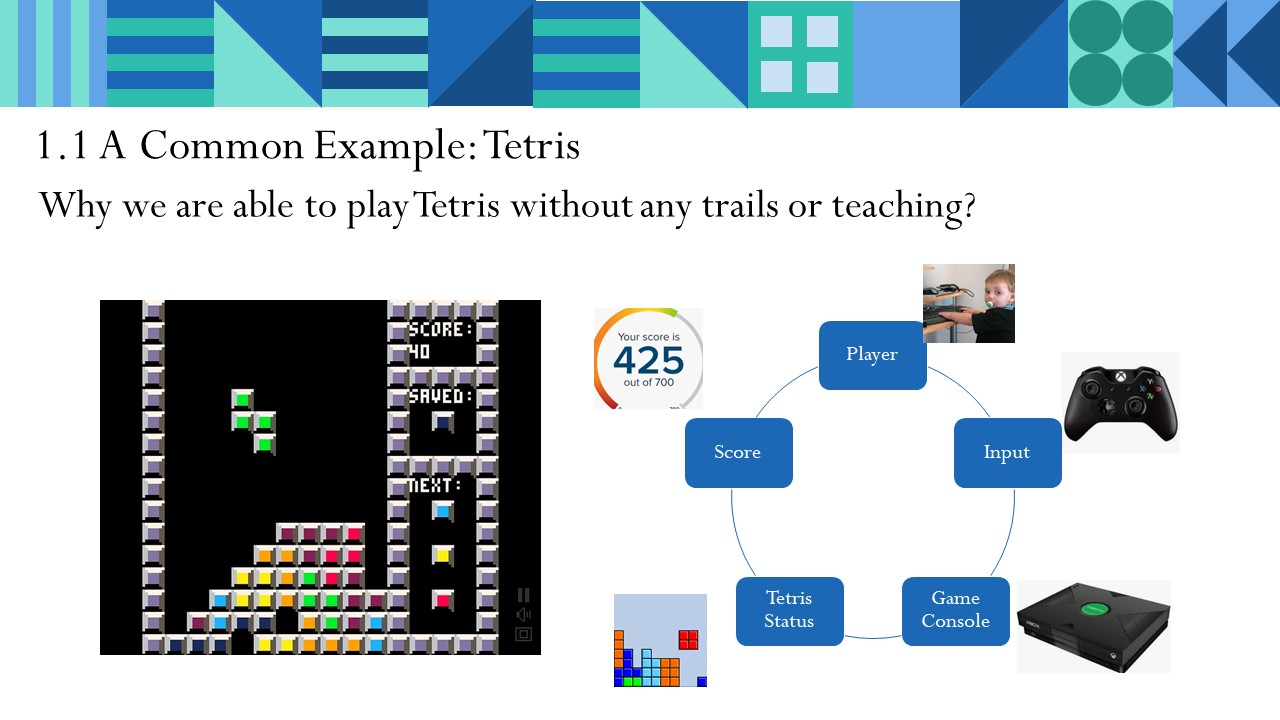• 玩家：游戏游玩者
• 玩家操作：按自己的意志操作，输入给游戏
• 游戏机：玩家面对的环境载体。
• 方块状态：让玩家获知现在的方块堆叠状态
• 特效(爆炸消除/游戏结束)：给予玩家的反馈

• 玩家：Agent。可以翻译为“智能体”，一般是场景中行动主体
• 玩家操作：Action行动
• 游戏机：Environment，环境
• 方块状态：Observation，观察值，反馈给Agent。
• 特效(爆炸消除/游戏结束)：Reward反馈。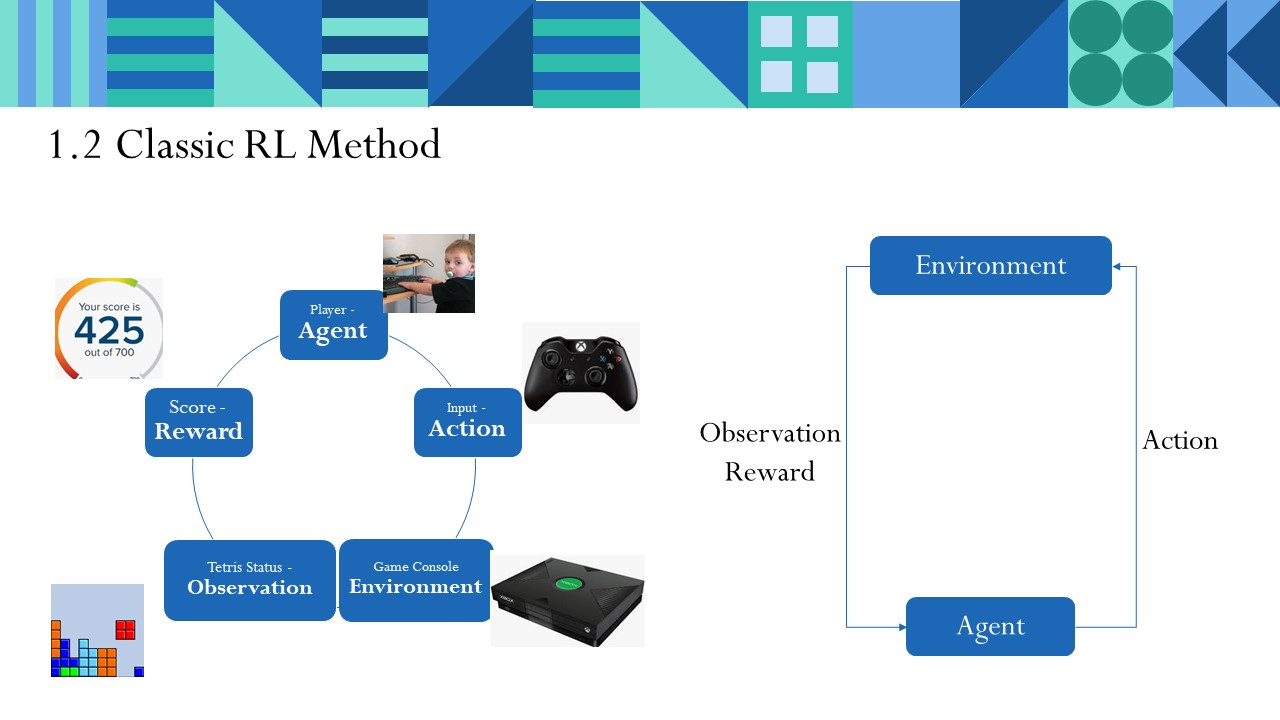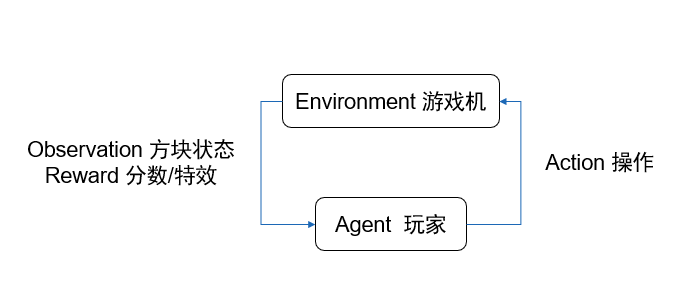### 1.3 强化学习的特点和评价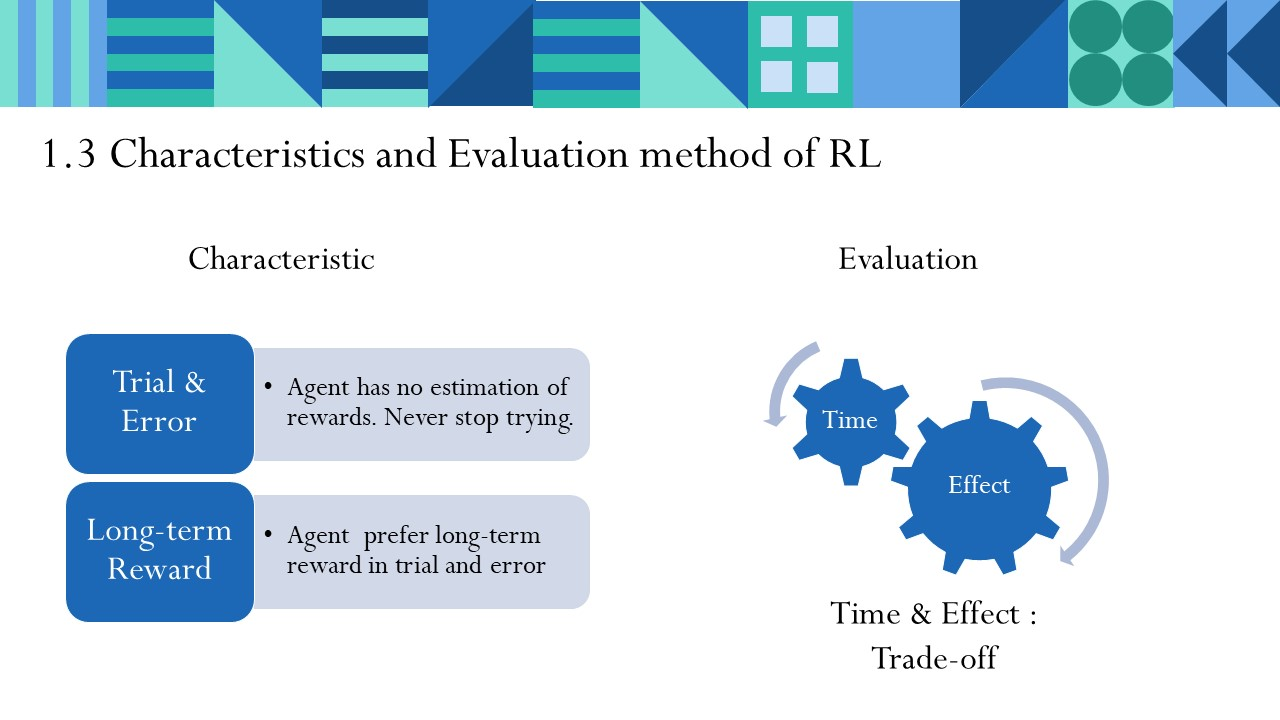Agent不知道自己给出行动会收到怎样的奖励，因此Agent必须不断尝试（Trial & Error），才能得到成功。

Agent在不断试错之中，更加看中长时间尺度内能获得的回报，即使会遇到更多失败挫折。

## 2 Q-Learning

### 2.1 Q-Learning决策

•$a_1$ 一下消除4行！结果爽到！！
•$a_2$ 一下消除1行，感觉还行。
•$a_3$ 一行都没消除！你在逗我？距离Gameover又近了一步！Q表：描述我在所有状态中所有行动的数值矩阵，我不管，我就在Q表中选这其中的最大值行动。

### 2.2 Q-Learning 更新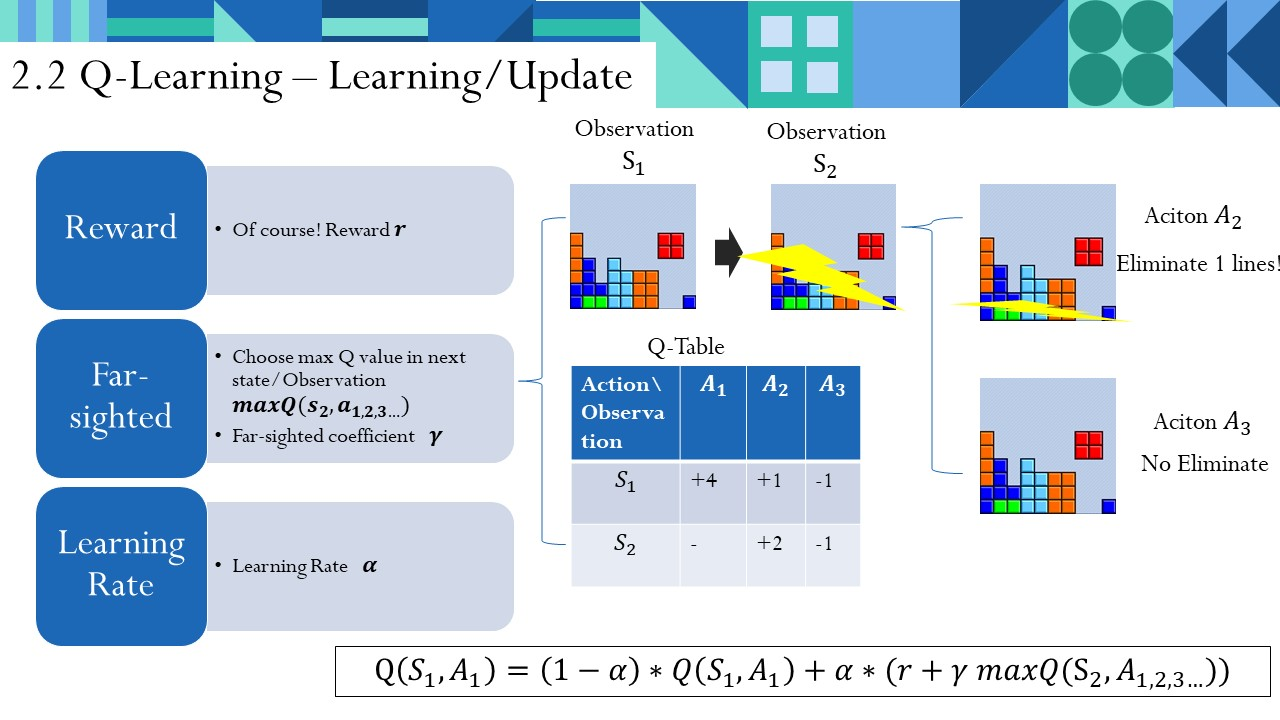$Q(S_1,A_1) = (1-\alpha)*Q(S_1,A_1)+\alpha*(r+\gamma maxQ(s_2,a_{1,2,3...}))$

### 2.3 Q-Learning算法流程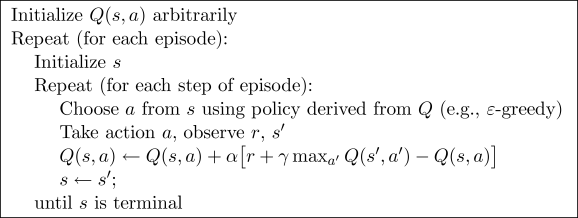Q-Learning算法流程如上图。在一定的episode里，我不断地重复、试错，更新Q表，直到某个时间点收敛。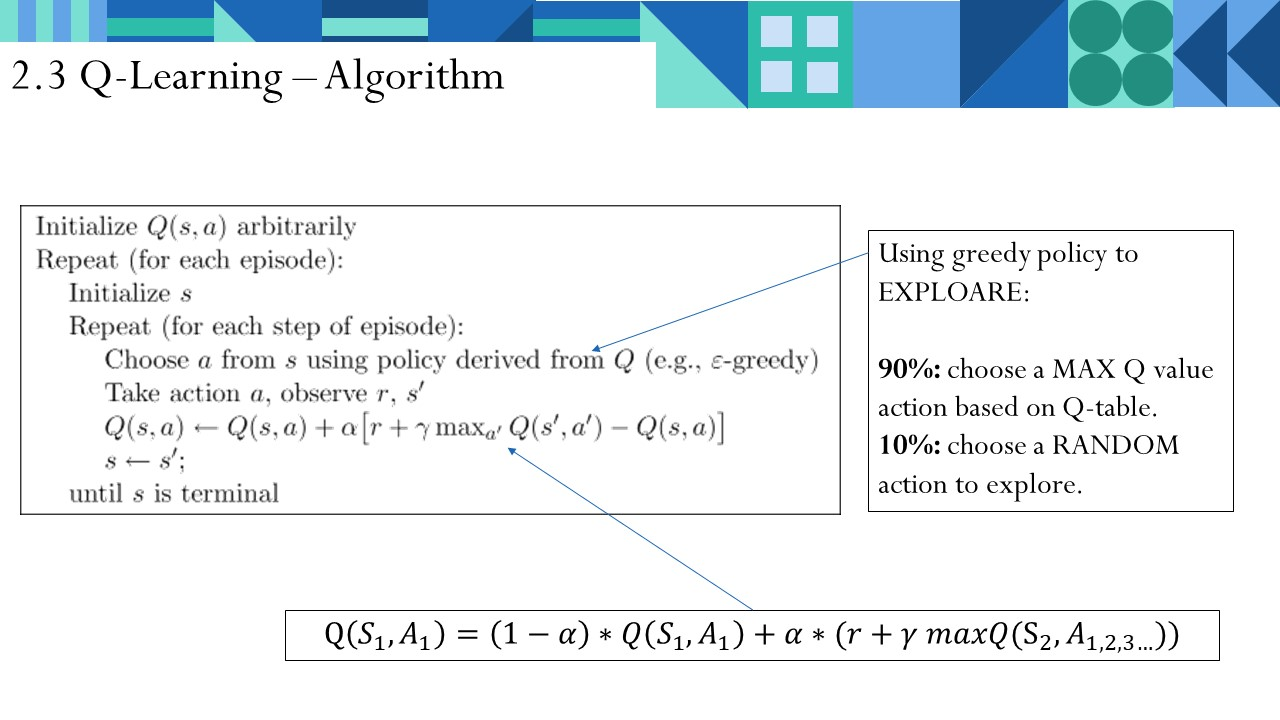### 2.4 Q-Learning性质

• 无环境模型 – QL不理解所处环境，没有对环境进行建模。是根据环境反馈，学习做出决策。
• 单步更新 – QL在每走一步就更新Q表。有的算法是回合结束后更新。

## 3 实验

https://morvanzhou.github.io/tutorials/machine-learning/reinforcement-learning/2-2-tabular-q1/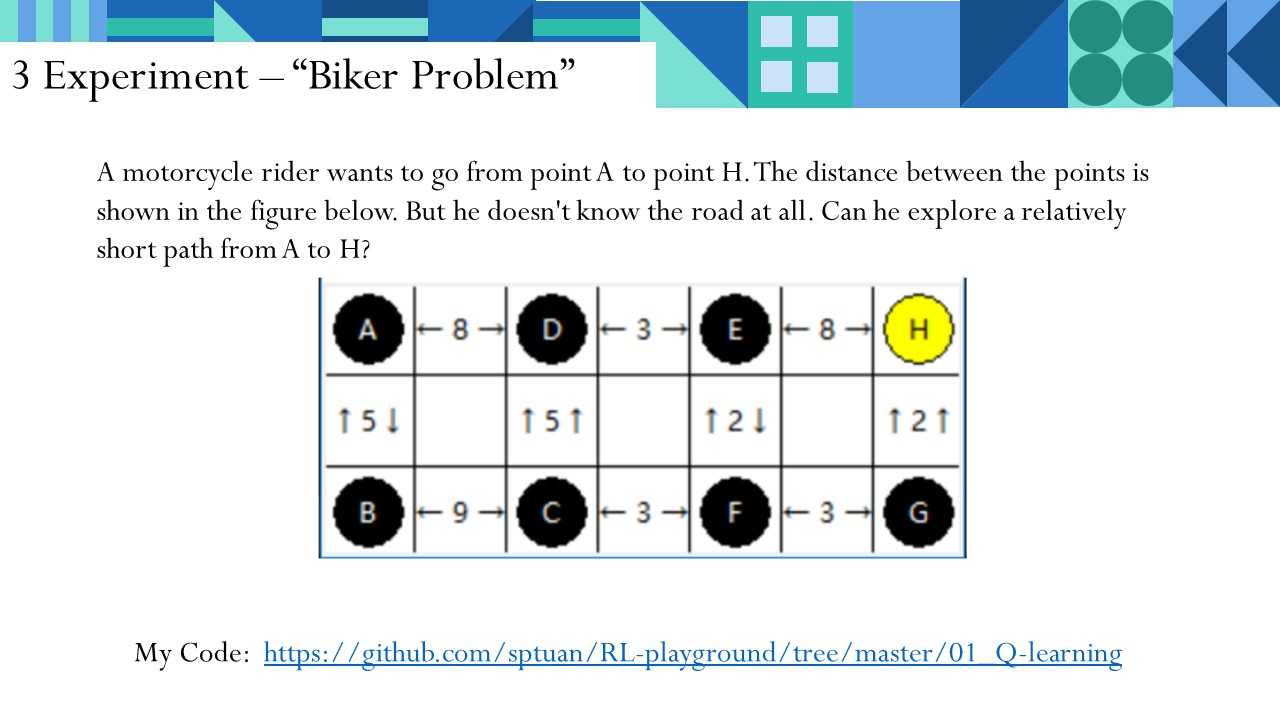https://github.com/sptuan/RL-playground/tree/master/01_Q-learning/ex2

### 3.1 问题描述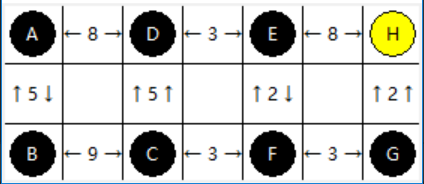### 3.2 问题抽象

env_tk.py

RL_brain.py

"""
Reinforcement learning maze example.
This script is our Q-Learning brain.
This script is modified from https://morvanzhou.github.io/tutorials/
"""

import numpy as np
import pandas as pd
import os

class QLearningTable:
def __init__(self, actions, learning_rate=0.01, reward_decay=0.9, e_greedy=0.9):
self.actions = actions  # a list
self.lr = learning_rate
self.gamma = reward_decay
self.epsilon = e_greedy
self.q_table = pd.DataFrame(columns=self.actions, dtype=np.float64)

def choose_action(self, observation):
self.check_state_exist(observation)
# action selection
if np.random.uniform() < self.epsilon:
# choose best action
state_action = self.q_table.loc[observation, :]
#state_action = state_action.reindex(np.random.permutation(state_action.index))     # some actions have same value
action = state_action.idxmax()
else:
# choose random action
action = np.random.choice(self.actions)
return action

def learn(self, s, a, r, s_):
self.check_state_exist(s_)
q_predict = self.q_table.loc[s, a]
if s_ != 'H1':
q_target = r + self.gamma * self.q_table.loc[s_, :].max()  # next state is not terminal
else:
q_target = r  # next state is terminal
self.q_table.loc[s, a] += self.lr * (q_target - q_predict)  # update
os.system('cls')
print(self.q_table)

def check_state_exist(self, state):
if state not in self.q_table.index:
# append new state to q table
self.q_table = self.q_table.append(
pd.Series(
*len(self.actions),
index=self.q_table.columns,
name=state,
)
)

main.py

"""
Reinforcement learning maze example.
This script is our main script, in which a bike driver tries to arrive at FINAL POINT.
This script is modified from https://morvanzhou.github.io/tutorials/
"""

from env_tk import Maze
from RL_brain import QLearningTable

def update():
for episode in range(10000):
# initial observation
# return state, format "A B C ..."
action_space = env.action_space
observation = env.reset()

while True:
# fresh env, tkinter
env.render()

# RL choose action based on observation

#while True:
action = RL.choose_action(str(observation))
if action == 0:
action_step = 'UP'
elif action == 1:
action_step = 'DOWN'
elif action == 2:
action_step = 'RIGHT'
elif action == 3:
action_step = 'LEFT'
#if action_step in action_space:
#   break

# RL take action and get next observation and reward
observation_, reward, done, action_space = env.step(action_step)

# RL learn from this transition
RL.learn(str(observation), action, reward, str(observation_))

# swap observation
observation = observation_

# break while loop when end of this episode
if done:
break

# end of game
print('game over')
env.destroy()

if __name__ == "__main__":
env = Maze()
RL = QLearningTable(actions=list(range(4)))

env.after(50, update)
env.mainloop()

### 3.3 实验结果

• 成功找到了终点。
• 但是结果不是最优解，陷入了局部最优解中。

## 5参考资料

 莫凡的强化学习教程 https://morvanzhou.github.io/tutorials/machine-learning/reinforcement-learning/

 Reinforcement Learning: An Introduction.  Richard S. Sutton and Andrew G. Barto

0 0 vote
Article Rating
Subscribe9 评论
Inline Feedbacks2 年 之前2 年 之前xywang
2 年 之前2 年 之前John Raymond
2 年 之前

impppppppport